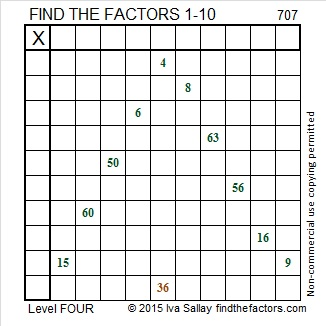# 707 and Level 4

• 707 is a composite number.
• Prime factorization: 707 = 7 x 101
• The exponents in the prime factorization are 1 and 1. Adding one to each and multiplying we get (1 + 1)(1 + 1) = 2 x 2 = 4. Therefore 707 has exactly 4 factors.
• Factors of 707: 1, 7, 101, 707
• Factor pairs: 707 = 1 x 707 or 7 x 101
• 707 has no square factors that allow its square root to be simplified. √707 ≈ 26.58947.Here is today’s factoring puzzle:Print the puzzles or type the solution on this excel file: 10 Factors 2015-12-07

————————————————————————————

Here are a few more facts about 707:

707 is a palindrome in base 10 and in base 100.

707 is the sum of seven consecutive numbers: 98 + 99 + 100 + 101 + 102 + 103 + 104 = 707

It is also the sum of two consecutive numbers: 353 + 354 = 707.

707 is the sum of these five consecutive prime numbers: 131 + 137 + 139 + 149 + 151 = 707.

And it is the sum of the nineteen prime numbers from 5 to 73.

Since 101 is one of its prime factors, 707 is the hypotenuse of Pythagorean triple 140-693-707. What is the greatest common factor of those three numbers?

————————————————————————————